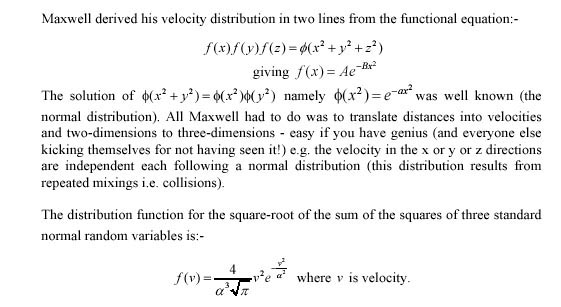The density function, f(v), for the distribution of the velocity of molecules in a gas, is the distribution of the square-root of a chi-squred distribution with three degrees of freedom as it is the square-root of the sum of three independent random variables (the velocities in the three mutually perpendicular directions) each of which is chi-squared with one degree of freedom (each being independently distributed as the square of normal distributions).

Maxwell derived his velocity distribution in two lines from the functional equation: